# Coriolis effect

﻿
Coriolis effectFigure 1: In the inertial frame of reference (upper part of the picture), the black object moves in a straight line, without significant friction with the disc. However, the observer (red dot) who is standing in the rotating (non-inertial) frame of reference (lower part of the picture) sees the object as following a curved path due to the Coriolis and centrifugal forces present in this frame.

In physics, the Coriolis effect is a deflection of moving objects when they are viewed in a rotating reference frame. In a reference frame with clockwise rotation, the deflection is to the left of the motion of the object; in one with counter-clockwise rotation, the deflection is to the right. The mathematical expression for the Coriolis force appeared in an 1835 paper by French scientist Gaspard-Gustave Coriolis, in connection with the theory of water wheels, and also in the tidal equations of Pierre-Simon Laplace in 1778. And even earlier, Italian scientists Giovanni Battista Riccioli and his assistant Francesco Maria Grimaldi described the effect in connection with artillery in the 1651 Almagestum Novum, writing that rotation of the Earth should cause a cannon ball fired to the north to deflect to the east. Early in the 20th century, the term Coriolis force began to be used in connection with meteorology.

The Coriolis effect is caused by the rotation of the Earth and the inertia of the mass experiencing the effect. Newton's laws of motion govern the motion of an object in a (non-accelerating) inertial frame of reference. When Newton's laws are transformed to a rotating frame of reference, the Coriolis and centrifugal forces appear. Both forces are proportional to the mass of the object. The Coriolis force is proportional to the rotation rate and the centrifugal force is proportional to its square. The Coriolis force acts in a direction perpendicular to the rotation axis and to the velocity of the body in the rotating frame and is proportional to the object's speed in the rotating frame. The centrifugal force acts outwards in the radial direction and is proportional to the distance of the body from the axis of the rotating frame. These additional forces are termed either inertial forces, fictitious forces or pseudo forces. They allow the application of simple Newtonian laws to a rotating system. They are correction factors that do not exist in a true non-accelerating "inertial" system.

Perhaps the most commonly encountered rotating reference frame is the Earth. Because the Earth completes only one rotation per day, the Coriolis force is quite small, and its effects generally become noticeable only for motions occurring over large distances and long periods of time, such as large-scale movement of air in the atmosphere or water in the ocean. Such motions are constrained by the 2-dimensional surface of the earth, so only the horizontal component of the Coriolis force is generally important. This force causes moving objects on the surface of the Earth to appear to veer to the right in the northern hemisphere, and to the left in the southern. Rather than flowing directly from areas of high pressure to low pressure, as they would on a non-rotating planet, winds and currents tend to flow to the right of this direction north of the equator, and to the left of this direction south of it. This effect is responsible for the rotation of large cyclones (see Coriolis effects in meteorology).

## History

Gaspard-Gustave Coriolis published a paper in 1835 on the energy yield of machines with rotating parts, such as waterwheels. That paper considered the supplementary forces that are detected in a rotating frame of reference. Coriolis divided these supplementary forces into two categories. The second category contained a force that arises from the cross product of the angular velocity of a coordinate system and the projection of a particle's velocity into a plane perpendicular to the system's axis of rotation. Coriolis referred to this force as the "compound centrifugal force" due to its analogies with the centrifugal force already considered in category one. By the early 20th century the effect was known as the "acceleration of Coriolis". By 1919 it was referred to as "Coriolis' force" and by 1920 as "Coriolis force".

In 1856, William Ferrel proposed the existence of a circulation cell in the mid-latitudes with air being deflected by the Coriolis force to create the prevailing westerly winds.

Understanding the kinematics of how exactly the rotation of the Earth affects airflow was partial at first. Late in the 19th century, the full extent of the large scale interaction of pressure gradient force and deflecting force that in the end causes air masses to move 'along' isobars was understood.[citation needed]

## Formula

In non-vector terms: at a given rate of rotation of the observer, the magnitude of the Coriolis acceleration of the object is proportional to the velocity of the object and also to the sine of the angle between the direction of movement of the object and the axis of rotation.

The vector formula for the magnitude and direction of the Coriolis acceleration is$\boldsymbol{ a}_C = -2 \, \boldsymbol{ \Omega \times v}$

where (here and below)$\boldsymbol{ a}_C$ is the acceleration of the particle in the rotating system,$\boldsymbol{v}\,$ is the velocity of the particle in the rotating system, and Ω is the angular velocity vector which has magnitude equal to the rotation rate ω and is directed along the axis of rotation of the rotating reference frame, and the × symbol represents the cross product operator.

The equation may be multiplied by the mass of the relevant object to produce the Coriolis force:$\boldsymbol{ F}_C = -2 \, m \, \boldsymbol{\Omega \times v}$.

See fictitious force for a derivation.

The Coriolis effect is the behavior added by the Coriolis acceleration. The formula implies that the Coriolis acceleration is perpendicular both to the direction of the velocity of the moving mass and to the frame's rotation axis. So in particular:

• if the velocity is parallel to the rotation axis, the Coriolis acceleration is zero.
• if the velocity is straight inward to the axis, the acceleration is in the direction of local rotation.
• if the velocity is straight outward from the axis, the acceleration is against the direction of local rotation.
• if the velocity is in the direction of local rotation, the acceleration is outward from the axis.
• if the velocity is against the direction of local rotation, the acceleration is inward to the axis.

The vector cross product can be evaluated as the determinant of a matrix:$\boldsymbol{\Omega \times v} = \begin{vmatrix} \boldsymbol{i}&\boldsymbol{j}&\boldsymbol{k} \\ \Omega_x & \Omega_y & \Omega_z \\ v_x & v_y & v_z \end{vmatrix}\ = \begin{pmatrix} \Omega_y v_z - \Omega_z v_y \\ \Omega_z v_x - \Omega_x v_z \\ \Omega_x v_y - \Omega_y v_x \end{pmatrix}\ ,$

where the vectors i, j, k are unit vectors in the x, y and z directions.

## Causes

The Coriolis effect exists only when one uses a rotating reference frame. In the rotating frame it behaves exactly like a real force (that is to say, it causes acceleration and has real effects). However, Coriolis force is a consequence of inertia, and is not attributable to an identifiable originating body, as is the case for electromagnetic or nuclear forces, for example. From an analytical viewpoint, to use Newton's second law in a rotating system, Coriolis force is mathematically necessary, but it disappears in a non-accelerating, inertial frame of reference. For example, consider two children on opposite sides of a spinning roundabout (carousel), who are throwing a ball to each other (see Figure 1). From the children's point of view, this ball's path is curved sideways by the Coriolis effect. Suppose the roundabout spins counter-clockwise when viewed from above. From the thrower's perspective, the deflection is to the right. From the non-thrower's perspective, deflection is to left. For a mathematical formulation see Mathematical derivation of fictitious forces.

A denizen of a rotating frame, such as an astronaut in a rotating space station, very probably will find the interpretation of everyday life in terms of the Coriolis force accords more simply with intuition and experience than a cerebral reinterpretation of events from an inertial standpoint. For example, nausea due to an experienced push may be more instinctively explained by Coriolis force than by the law of inertia. See also Coriolis effect (perception). In meteorology, a rotating frame (the Earth) with its Coriolis force proves a more natural framework for explanation of air movements than a hypothetical, non-rotating, inertial frame without Coriolis forces. In long-range gunnery, sight corrections for the Earth's rotation are based upon Coriolis force. These examples are described in more detail below.

The acceleration entering the Coriolis force arises from two sources of change in velocity that result from rotation: the first is the change of the velocity of an object in time. The same velocity (in an inertial frame of reference where the normal laws of physics apply) will be seen as different velocities at different times in a rotating frame of reference. The apparent acceleration is proportional to the angular velocity of the reference frame (the rate at which the coordinate axes change direction), and to the component of velocity of the object in a plane perpendicular to the axis of rotation. This gives a term$-\boldsymbol\Omega\times\boldsymbol v$. The minus sign arises from the traditional definition of the cross product (right hand rule), and from the sign convention for angular velocity vectors.

The second is the change of velocity in space. Different positions in a rotating frame of reference have different velocities (as seen from an inertial frame of reference). In order for an object to move in a straight line it must therefore be accelerated so that its velocity changes from point to point by the same amount as the velocities of the frame of reference. The effect is proportional to the angular velocity (which determines the relative speed of two different points in the rotating frame of reference), and to the component of the velocity of the object in a plane perpendicular to the axis of rotation (which determines how quickly it moves between those points). This also gives a term$-\boldsymbol\Omega\times\boldsymbol v$.

## Length scales and the Rossby number

The time, space and velocity scales are important in determining the importance of the Coriolis effect. Whether rotation is important in a system can be determined by its Rossby number, which is the ratio of the velocity, U, of a system to the product of the Coriolis parameter,$f = 2 \omega \sin \varphi \,$, and the length scale, L, of the motion:$Ro = \frac{U}{fL}$.

The Rossby number is the ratio of inertial to Coriolis forces. A small Rossby number signifies a system which is strongly affected by Coriolis forces, and a large Rossby number signifies a system in which inertial forces dominate. For example, in tornadoes, the Rossby number is large, in low-pressure systems it is low and in oceanic systems it is of the order of unity. As a result, in tornadoes the Coriolis force is negligible, and balance is between pressure and centrifugal forces. In low-pressure systems, centrifugal force is negligible and balance is between Coriolis and pressure forces. In the oceans all three forces are comparable.

An atmospheric system moving at U = 10 m/s occupying a spatial distance of L = 1,000 km (621 mi), has a Rossby number of approximately 0.1. A man playing catch may throw the ball at U = 30 m/s in a garden of length L = 50 m. The Rossby number in this case would be about = 6000. Needless to say, one does not worry about which hemisphere one is in when playing catch in the garden. However, an unguided missile obeys exactly the same physics as a baseball, but may travel far enough and be in the air long enough to notice the effect of Coriolis. Long-range shells in the Northern Hemisphere landed close to, but to the right of, where they were aimed until this was noted. (Those fired in the southern hemisphere landed to the left.) In fact, it was this effect that first got the attention of Coriolis himself.

## Applied to Earth

An important case where the Coriolis force is observed is the rotating Earth.

### Intuitive explanation

As the Earth turns around its axis, everything attached to it turns with it (imperceptibly to our senses). An object that is moving without being dragged along with this rotation travels in a straight motion over the turning Earth, seeming (from our rotating perspective upon the planet) to change its direction of motion as it moves, thus appearing to travel along a curved path that bends in the opposite direction to our actual motion and tracing out a path on the ground below that curves the same way. When viewed from a stationary point in space above, any land feature in the Northern Hemisphere turns counter-clockwise, and, fixing our gaze on that location, any other location in that hemisphere will rotate around it the same way. The traced ground-path of a freely moving body traveling from one point to another will therefore bend the opposite way, clockwise, which is conventionally labeled as "right," where it will be if the direction of motion is considered "ahead" and "down" is defined naturally.

### Rotating sphereFigure 2: Coordinate system at latitude φ with x-axis east, y-axis north and z-axis upward (that is, radially outward from center of sphere).

Consider a location with latitude φ on a sphere that is rotating around the north-south axis. A local coordinate system is set up with the x axis horizontally due east, the y axis horizontally due north and the z axis vertically upwards. The rotation vector, velocity of movement and Coriolis acceleration expressed in this local coordinate system (listing components in the order East (e), North (n) and Upward (u)) are:$\boldsymbol{ \Omega} = \omega \begin{pmatrix} 0 \\ \cos \varphi \\ \sin \varphi \end{pmatrix}\ ,$$\boldsymbol{ v} = \begin{pmatrix} v_e \\ v_n \\ v_u \end{pmatrix}\ ,$$\boldsymbol{ a}_C =-2\boldsymbol{\Omega \times v}= 2\,\omega\, \begin{pmatrix} v_n \sin \varphi-v_u \cos \varphi \\ -v_e \sin \varphi \\ v_e \cos\varphi\end{pmatrix}\ .$

When considering atmospheric or oceanic dynamics, the vertical velocity is small and the vertical component of the Coriolis acceleration is small compared to gravity. For such cases, only the horizontal (East and North) components matter. The restriction of the above to the horizontal plane is (setting vu=0):$\boldsymbol{ v} = \begin{pmatrix} v_e \\ v_n\end{pmatrix}\ ,$$\boldsymbol{ a}_c = \begin{pmatrix} v_n \\ -v_e\end{pmatrix}\ f\ ,$

where$f = 2 \omega \sin \varphi \,$ is called the Coriolis parameter.

By setting vn = 0, it can be seen immediately that (for positive φ and ω) a movement due east results in an acceleration due south. Similarly, setting ve = 0, it is seen that a movement due north results in an acceleration due east. In general, observed horizontally, looking along the direction of the movement causing the acceleration, the acceleration always is turned 90° to the right and of the same size regardless of the horizontal orientation. That is:

On a merry-go-round in the night
Coriolis was shaken with fright
Despite how he walked
'Twas like he was stalked
By some fiend always pushing him right

David Morin, Eric Zaslow, E'beth Haley, John Golden, and Nathan Salwen

As a different case, consider equatorial motion setting φ = 0°. In this case, Ω is parallel to the North or n-axis, and:$\boldsymbol{ \Omega} = \omega \begin{pmatrix} 0 \\ 1 \\ 0 \end{pmatrix}\ ,$$\boldsymbol{ v} = \begin{pmatrix} v_e \\ v_n \\ v_u \end{pmatrix}\ ,$$\boldsymbol{ a}_C =-2\boldsymbol{\Omega \times v}= 2\,\omega\, \begin{pmatrix}-v_u \\0 \\ v_e \end{pmatrix}\ .$

Accordingly, an eastward motion (that is, in the same direction as the rotation of the sphere) provides an upward acceleration known as the Eötvös effect, and an upward motion produces an acceleration due west.

### Distant stars

The apparent motion of a distant star as seen from Earth is dominated by the Coriolis and centrifugal forces. Consider such a star (with mass m) located at position r, with declination δ, so Ω · r = |r| Ω sin(δ), where Ω is the Earth's rotation vector. The star is observed to rotate about the Earth's axis with a period of one sidereal day in the opposite direction to that of the Earth's rotation, making its velocity v = –Ω × r. The fictitious force, consisting of Coriolis and centrifugal forces, is:$\boldsymbol {F_f} = -2 \, m \, \boldsymbol{\Omega \times v} - m \, \boldsymbol{\Omega \times { (\Omega \times r)}}$$= +2 \, m \, \boldsymbol{\Omega \times (\Omega \times r)} - m \, \boldsymbol{\Omega \times {(\Omega \times r)}}$$= m \, \boldsymbol{\Omega \times (\Omega \times r)}$$= m \, \boldsymbol{(\Omega (\Omega \cdot r)} - \boldsymbol{r (\Omega \cdot \Omega))}$$= - m \, \Omega^2 \, \boldsymbol{ ( r} - \mid\boldsymbol{r}\mid \sin(\delta)\boldsymbol{u_\Omega)}$,

where uΩ = Ω−1Ω is a unit vector in the direction of Ω. The fictitious force Ff is thus a vector of magnitude m Ω2|r| cos(δ), perpendicular to Ω, and directed towards the center of the star's rotation on the Earth's axis, and therefore recognisable as the centripetal force that will keep the star in a circular movement around that axis.

### Meteorology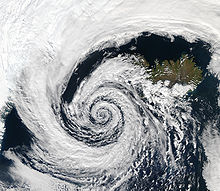Figure 12: This low pressure system over Iceland spins counter-clockwise due to balance between the Coriolis force and the pressure gradient force.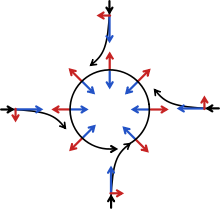Figure 13: Schematic representation of flow around a low-pressure area in the Northern hemisphere. The Rossby number is low, so the centrifugal force is virtually negligible. The pressure-gradient force is represented by blue arrows, the Coriolis acceleration (always perpendicular to the velocity) by red arrows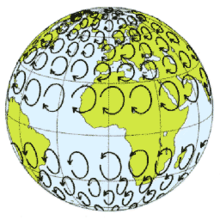Figure 14: Schematic representation of inertial circles of air masses in the absence of other forces, calculated for a wind speed of approximately 50 to 70 m/s. Note that the rotation is exactly opposite of that normally experienced with air masses in weather systems around depressions.

Perhaps the most important instance of the Coriolis effect is in the large-scale dynamics of the oceans and the atmosphere. In meteorology and oceanography, it is convenient to postulate a rotating frame of reference wherein the Earth is stationary. In accommodation of that provisional postulation, the otherwise fictitious centrifugal and Coriolis forces are introduced. Their relative importance is determined by the applicable Rossby numbers. Tornadoes have high Rossby numbers, so, while tornado-associated centrifugal forces are quite substantial, Coriolis forces associated with tornados are for practical purposes negligible.

High pressure systems rotate in a direction such that the Coriolis force will be directed radially inwards, and nearly balanced by the outwardly radial pressure gradient. This direction is clockwise in the northern hemisphere and counter-clockwise in the southern hemisphere. Low pressure systems rotate in the opposite direction, so that the Coriolis force is directed radially outward and nearly balances an inwardly radial pressure gradient. In each case a slight imbalance between the Coriolis force and the pressure gradient accounts for the radially inward acceleration of the system's circular motion.

#### Flow around a low-pressure area

If a low-pressure area forms in the atmosphere, air will tend to flow in towards it, but will be deflected perpendicular to its velocity by the Coriolis force. A system of equilibrium can then establish itself creating circular movement, or a cyclonic flow. Because the Rossby number is low, the force balance is largely between the pressure gradient force acting towards the low-pressure area and the Coriolis force acting away from the center of the low pressure.

Instead of flowing down the gradient, large scale motions in the atmosphere and ocean tend to occur perpendicular to the pressure gradient. This is known as geostrophic flow. On a non-rotating planet fluid would flow along the straightest possible line, quickly eliminating pressure gradients. Note that the geostrophic balance is thus very different from the case of "inertial motions" (see below) which explains why mid-latitude cyclones are larger by an order of magnitude than inertial circle flow would be.

This pattern of deflection, and the direction of movement, is called Buys-Ballot's law. In the atmosphere, the pattern of flow is called a cyclone. In the Northern Hemisphere the direction of movement around a low-pressure area is anticlockwise. In the Southern Hemisphere, the direction of movement is clockwise because the rotational dynamics is a mirror image there. At high altitudes, outward-spreading air rotates in the opposite direction. Cyclones rarely form along the equator due to the weak Coriolis effect present in this region.

#### Inertial circles

An air or water mass moving with speed$v\,$ subject only to the Coriolis force travels in a circular trajectory called an 'inertial circle'. Since the force is directed at right angles to the motion of the particle, it will move with a constant speed, and perform a complete circle with frequency f. The magnitude of the Coriolis force also determines the radius of this circle:$R= \frac {v}{2 \pi f}\,$

On the Earth, a typical mid-latitude value for f is 10−4 s−1; hence for a typical atmospheric speed of 10 m/s the radius is 100 km (62 mi), with a period of about 14 hours. For an ocean current with a typical speed of 10 cm/s, the radius of an inertial circle is 1 km (0.6 mi). These inertial circles are clockwise in the northern hemisphere (where trajectories are bent to the right) and anti-clockwise in the southern hemisphere.

If the rotating system is a parabolic turntable, then f is constant and the trajectories are exact circles. On a rotating planet, f varies with latitude and the paths of particles do not form exact circles. Since the parameter f varies as the sine of the latitude, the radius of the oscillations associated with a given speed are smallest at the poles (latitude = ±90°), and increase toward the equator.

#### Other terrestrial effects

The Coriolis effect strongly affects the large-scale oceanic and atmospheric circulation, leading to the formation of robust features like jet streams and western boundary currents. Such features are in geostrophic balance, meaning that the Coriolis and pressure gradient forces balance each other. Coriolis acceleration is also responsible for the propagation of many types of waves in the ocean and atmosphere, including Rossby waves and Kelvin waves. It is also instrumental in the so-called Ekman dynamics in the ocean, and in the establishment of the large-scale ocean flow pattern called the Sverdrup balance.

### Eötvös effect

The practical impact of the Coriolis effect is mostly caused by the horizontal acceleration component produced by horizontal motion.

There are other components of the Coriolis effect. Eastward-traveling objects will be deflected upwards (feel lighter), while westward-traveling objects will be deflected downwards (feel heavier). This is known as the Eötvös effect. This aspect of the Coriolis effect is greatest near the equator. The force produced by this effect is similar to the horizontal component, but the much larger vertical forces due to gravity and pressure mean that it is generally unimportant dynamically.

In addition, objects traveling upwards or downwards will be deflected to the west or east respectively. This effect is also the greatest near the equator. Since vertical movement is usually of limited extent and duration, the size of the effect is smaller and requires precise instruments to detect.

### Draining in bathtubs and toilets

In 1908, the Austrian physicist Otto Tumlirz described careful and effective experiments which demonstrated the effect of the rotation of the Earth on the outflow of water through a central aperture. The subject was later popularized in a famous article in the journal Nature, which described an experiment in which all other forces to the system were removed by filling a 6-foot (1.8 m) tank with 300 US gallons (1,100 l) of water and allowing it to settle for 24 hours (to allow any movement due to filling the tank to die away), in a room where the temperature had stabilized. The drain plug was then very slowly removed, and tiny pieces of floating wood were used to observe rotation. During the first 12 to 15 minutes, no rotation was observed. Then, a vortex appeared and consistently began to rotate in a counter-clockwise direction (the experiment was performed in Boston, Massachusetts, in the Northern hemisphere). This was repeated and the results averaged to make sure the effect was real. The report noted that the vortex rotated, "about 30,000 times faster than the effective rotation of the earth in 42° North (the experiment's location)". This shows that the small initial rotation due to the earth is amplified by gravitational draining and conservation of angular momentum to become a rapid vortex and may be observed under carefully controlled laboratory conditions.

The formation of a vortex over the plug hole may be explained by the conservation of angular momentum. The radius of rotation decreases as water approaches the plug hole so the rate of rotation increases, for the same reason that an ice skater's rate of spin increases as she pulls her arms in. Any rotation around the plug hole that is initially present accelerates as water moves inward. If the water is so still that the effective rotation rate of the earth (once per day at the poles, once every 2 days at 30 degrees of latitude) is faster than that of the water relative to its container, and if externally applied torques (such as might be caused by flow over an uneven bottom surface) are small enough, the Coriolis effect may determine the direction of the vortex. Without such careful preparation, the Coriolis effect may be much smaller than various other influences on drain direction, such as any residual rotation of the water and the geometry of the container. Despite this, the idea that toilets and bathtubs drain differently in the Northern and Southern Hemispheres has been popularized by several television programs, including The Simpsons episode "Bart vs. Australia" and The X-Files episode "Die Hand Die Verletzt". Several science broadcasts and publications, including at least one college-level physics textbook, have also stated this.

### Ballistic missiles and satellites

Ballistic missiles and satellites appear to follow curved paths when plotted on common world maps mainly because the Earth is spherical and the shortest distance between two points on the Earth's surface (called a great circle) is usually not a straight line on those maps. Every two-dimensional (flat) map necessarily distorts the Earth's curved (three-dimensional) surface. Typically (as in the commonly used Mercator projection, for example), this distortion increases with proximity to the poles. In the northern hemisphere for example, a ballistic missile fired toward a distant target using the shortest possible route (a great circle) will appear on such maps to follow a path north of the straight line from target to destination, and then curve back toward the equator. This occurs because the latitudes, which are projected as straight horizontal lines on most world maps, are in fact circles on the surface of a sphere, which get smaller as they get closer to the pole. Being simply a consequence of the sphericity of the Earth, this would be true even if the Earth didn't rotate. The Coriolis effect is of course also present, but its effect on the plotted path is much smaller.

The Coriolis effects became important in external ballistics for calculating the trajectories of very long-range artillery shells. The most famous historical example was the Paris gun, used by the Germans during World War I to bombard Paris from a range of about 120 km (75 mi).

## Special cases

### Cannon on turntable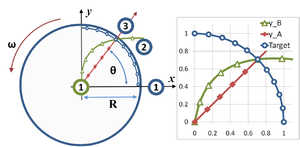Figure 3: Cannon at the centre of a rotating turntable. To hit the target located at position 1 on the perimeter at time t = 0s, the cannon must be aimed ahead of the target at angle θ. That way, by the time the cannonball reaches position 3 on the periphery, the target also will be at that position. In an inertial frame of reference, the cannonball travels a straight radial path to the target (curve yA). However, in the frame of the turntable, the path is arched (curve yB), as also shown in the figure.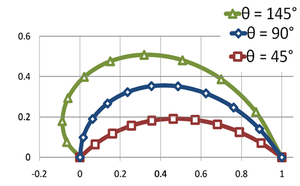Figure 4: Successful trajectory of cannonball as seen from the turntable for three angles of launch θ. Plotted points are for the same equally spaced times steps on each curve. Cannonball speed v is held constant and angular rate of rotation ω is varied to achieve a successful "hit" for selected θ. For example, for a radius of 1 m and a cannonball speed of 1 m/s, the time of flight tf = 1 s, and ωtf = θ → ω and θ have the same numerical value if θ is expressed in radians. The wider spacing of the plotted points as the target is approached show the speed of the cannonball is accelerating as seen on the turntable, due to fictitious Coriolis and centrifugal forces.Figure 5: Acceleration components at an earlier time (top) and at arrival time at the target (bottom)Figure 6: Coriolis acceleration, centrifugal acceleration and net acceleration vectors at three selected points on the trajectory as seen on the turntable.

Figure 1 is an animation of the classic illustration of Coriolis force. Another visualization of the Coriolis and centrifugal forces is this animation clip. Figure 3 is a graphical version.

Here is a question: given the radius of the turntable R, the rate of angular rotation ω, and the speed of the cannonball (assumed constant) v, what is the correct angle θ to aim so as to hit the target at the edge of the turntable?

The inertial frame of reference provides one way to handle the question: calculate the time to interception, which is tf = R / v . Then, the turntable revolves an angle ω tf in this time. If the cannon is pointed an angle θ = ω tf = ω R / v, then the cannonball arrives at the periphery at position number 3 at the same time as the target.

No discussion of Coriolis force can arrive at this solution as simply, so the reason to treat this problem is to demonstrate Coriolis formalism in an easily visualized situation.

The trajectory in the inertial frame (denoted A) is a straight line radial path at angle θ. The position of the cannonball in (x, y) coordinates at time t is:$\mathbf{r}_A (t)$$= vt\ \left( \cos (\theta ),\right.$$\left. {\color{white}..}\ \sin (\theta )\right) \ .$

In the turntable frame (denoted B), the x- y axes rotate at angular rate ω, so the trajectory becomes:$\mathbf{r}_B (t)$$= vt\ \left( \cos ( \theta - \omega t),\right.$$\left. {\color{white}..} \sin ( \theta - \omega t)\right) \ ,$

and three examples of this result are plotted in Figure 4.

To determine the components of acceleration, a general expression is used from the article fictitious force:$\mathbf{a}_{B} = \mathbf{a}_A$$- 2 \boldsymbol\Omega \times \mathbf{v}_{B}$$- \boldsymbol\Omega \times (\boldsymbol\Omega \times \mathbf{r}_B )$$- \frac{d \boldsymbol\Omega}{dt} \times \mathbf{r}_B \ ,$

in which the term in Ω × vB is the Coriolis acceleration and the term in Ω × ( Ω × rB) is the centrifugal acceleration. The results are (let α = θ − ωt):$\boldsymbol{\Omega} \mathbf{\times r_B}$$= \begin{vmatrix} \boldsymbol{i}&\boldsymbol{j}&\boldsymbol{k} \\ 0 & 0 & \omega \\ v t \cos \alpha & vt \sin \alpha & 0 \end{vmatrix}\$$= \omega t v \left(-\sin\alpha, \cos\alpha\right )\ ,$$\boldsymbol{\Omega \ \times} \left( \boldsymbol{\Omega} \mathbf{\times r_B}\right)$$= \begin{vmatrix} \boldsymbol{i}&\boldsymbol{j}&\boldsymbol{k} \\ 0 & 0 & \omega \\ -\omega t v \sin\alpha & \omega t v \cos\alpha & 0 \end{vmatrix}\ \ ,$

producing a centrifugal acceleration:$\mathbf{a_{\mathrm{Cfgl}}}$$= \omega^2 v t \left(\cos\alpha, \sin\alpha\right )=\omega^2 \mathbf{r_B}(t) \ .$

Also:$\mathbf{v_B} = \frac{d\mathbf{r_B}(t)}{dt}=(v \cos \alpha + \omega t \ v \sin \alpha,$$\ v \sin \alpha -\omega t \ v \cos \alpha ,\ 0)\ \ ,$$\boldsymbol{\Omega} \mathbf{\times v_B}$$= \begin{vmatrix}\! \boldsymbol{i}& \! \boldsymbol{j}& \! \boldsymbol{k} \\ 0 & 0 & \omega \\v \cos \alpha\quad &v \sin \alpha\quad &\quad \\ \; + \omega t \ v \sin \alpha & \; -\omega t \ v \cos \alpha & 0 \end{vmatrix}\ \ ,$

producing a Coriolis acceleration:$\mathbf{a_{\mathrm{Cor}}}$$= -2\left[ -\omega v \left( \sin\alpha - \omega t \cos\alpha\right),\right.$$\left. {\color{white}...}\ \omega v \left(\cos\alpha + \omega t \sin \alpha \right) \right]\$$=2\omega v \left(\sin\alpha,\ - \cos\alpha \right)$$-2\omega^2 \mathbf{r_B}(t) \ .$

Figure 5 and Figure 6 show these accelerations for a particular example.

It is seen that the Coriolis acceleration not only cancels the centrifugal acceleration, but together they provide a net "centripetal", radially inward component of acceleration (that is, directed toward the centre of rotation):$\mathbf{a_{\mathrm{Cptl}}} = -\omega^2 \mathbf{r_B}(t) \ ,$

and an additional component of acceleration perpendicular to rB (t):$\mathbf{a_{C\perp}}$$= 2\omega v \left(\sin\alpha,\ -\cos\alpha \right) \ .$

The "centripetal" component of acceleration resembles that for circular motion at radius rB, while the perpendicular component is velocity dependent, increasing with the radial velocity v and directed to the right of the velocity. The situation could be described as a circular motion combined with an "apparent Coriolis acceleration" of 2ωv. However, this is a rough labelling: a careful designation of the true centripetal force refers to a local reference frame that employs the directions normal and tangential to the path, not coordinates referred to the axis of rotation.

These results also can be obtained directly by two time differentiations of rB (t). Agreement of the two approaches demonstrates that one could start from the general expression for fictitious acceleration above and derive the trajectories of Figure 4. However, working from the acceleration to the trajectory is more complicated than the reverse procedure used here, which, of course, is made possible in this example by knowing the answer in advance.

As a result of this analysis an important point appears: all the fictitious accelerations must be included to obtain the correct trajectory. In particular, besides the Coriolis acceleration, the centrifugal force plays an essential role. It is easy to get the impression from verbal discussions of the cannonball problem, which are focussed on displaying the Coriolis effect particularly, that the Coriolis force is the only factor that must be considered; emphatically, that is not so. A turntable for which the Coriolis force is the only factor is the parabolic turntable. A somewhat more complex situation is the idealized example of flight routes over long distances, where the centrifugal force of the path and aeronautical lift are countered by gravitational attraction.

### Tossed ball on a rotating carousel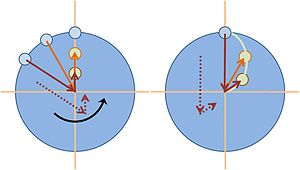Figure 7: A carousel is rotating anticlockwise. Left panel: a ball is tossed by a thrower at 12:00 o'clock and travels in a straight line to the centre of the carousel. While it travels, the thrower circles in an anticlockwise direction. Right panel: The ball's motion as seen by the thrower, who now remains at 12:00 o'clock, because there is no rotation from their viewpoint.

Figure 7 illustrates a ball tossed from 12:00 o'clock toward the centre of an anticlockwise rotating carousel. On the left, the ball is seen by a stationary observer above the carousel, and the ball travels in a straight line to the centre, while the ball-thrower rotates anticlockwise with the carousel. On the right the ball is seen by an observer rotating with the carousel, so the ball-thrower appears to stay at 12:00 o'clock. The figure shows how the trajectory of the ball as seen by the rotating observer can be constructed.

On the left, two arrows locate the ball relative to the ball-thrower. One of these arrows is from the thrower to the centre of the carousel (providing the ball-thrower's line of sight), and the other points from the centre of the carousel to the ball.(This arrow gets shorter as the ball approaches the centre.) A shifted version of the two arrows is shown dotted.

On the right is shown this same dotted pair of arrows, but now the pair are rigidly rotated so the arrow corresponding to the line of sight of the ball-thrower toward the centre of the carousel is aligned with 12:00 o'clock. The other arrow of the pair locates the ball relative to the centre of the carousel, providing the position of the ball as seen by the rotating observer. By following this procedure for several positions, the trajectory in the rotating frame of reference is established as shown by the curved path in the right-hand panel.

The ball travels in the air, and there is no net force upon it. To the stationary observer the ball follows a straight-line path, so there is no problem squaring this trajectory with zero net force. However, the rotating observer sees a curved path. Kinematics insists that a force (pushing to the right of the instantaneous direction of travel for an anticlockwise rotation) must be present to cause this curvature, so the rotating observer is forced to invoke a combination of centrifugal and Coriolis forces to provide the net force required to cause the curved trajectory.

### Bounced ball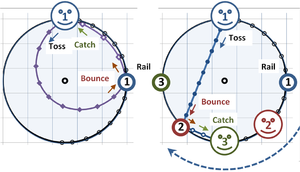Figure 8: Bird's-eye view of carousel. The carousel rotates clockwise. Two viewpoints are illustrated: that of the camera at the center of rotation rotating with the carousel (left panel) and that of the inertial (stationary) observer (right panel). Both observers agree at any given time just how far the ball is from the center of the carousel, but not on its orientation. Time intervals are 1/10 of time from launch to bounce.

Figure 8 describes a more complex situation where the tossed ball on a turntable bounces off the edge of the carousel and then returns to the tosser, who catches the ball. The effect of Coriolis force on its trajectory is shown again as seen by two observers: an observer (referred to as the "camera") that rotates with the carousel, and an inertial observer. Figure 8 shows a bird's-eye view based upon the same ball speed on forward and return paths. Within each circle, plotted dots show the same time points. In the left panel, from the camera's viewpoint at the center of rotation, the tosser (smiley face) and the rail both are at fixed locations, and the ball makes a very considerable arc on its travel toward the rail, and takes a more direct route on the way back. From the ball tosser's viewpoint, the ball seems to return more quickly than it went (because the tosser is rotating toward the ball on the return flight).

On the carousel, instead of tossing the ball straight at a rail to bounce back, the tosser must throw the ball toward the right of the target and the ball then seems to the camera to bear continuously to the left of its direction of travel to hit the rail (left because the carousel is turning clockwise). The ball appears to bear to the left from direction of travel on both inward and return trajectories. The curved path demands this observer to recognize a leftward net force on the ball. (This force is "fictitious" because it disappears for a stationary observer, as is discussed shortly.) For some angles of launch, a path has portions where the trajectory is approximately radial, and Coriolis force is primarily responsible for the apparent deflection of the ball (centrifugal force is radial from the center of rotation, and causes little deflection on these segments). When a path curves away from radial, however, centrifugal force contributes significantly to deflection.

The ball's path through the air is straight when viewed by observers standing on the ground (right panel). In the right panel (stationary observer), the ball tosser (smiley face) is at 12 o'clock and the rail the ball bounces from is at position one (1). From the inertial viewer's standpoint, positions one (1), two (2), three (3) are occupied in sequence. At position 2 the ball strikes the rail, and at position 3 the ball returns to the tosser. Straight-line paths are followed because the ball is in free flight, so this observer requires that no net force is applied.

## Visualization of the Coriolis effectFigure 9: A fluid assuming a parabolic shape as it is rotating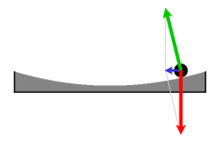Figure 10: The forces at play in the case of a curved surface.
Red: gravity
Green: the normal force
Blue: the resultant centripetal force.

To demonstrate the Coriolis effect, a parabolic turntable can be used. On a flat turntable, the inertia of a co-rotating object would force it off the edge. But if the surface of the turntable has the correct parabolic bowl shape (see Figure 9) and is rotated at the correct rate, the force components shown in Figure 10 are arranged so the component of gravity tangential to the bowl surface will exactly equal the centripetal force necessary to keep the object rotating at its velocity and radius of curvature (assuming no friction). (See banked turn.) This carefully contoured surface allows the Coriolis force to be displayed in isolation.

Discs cut from cylinders of dry ice can be used as pucks, moving around almost frictionlessly over the surface of the parabolic turntable, allowing effects of Coriolis on dynamic phenomena to show themselves. To get a view of the motions as seen from the reference frame rotating with the turntable, a video camera is attached to the turntable so as to co-rotate with the turntable, with results as shown in Figure 11. In the left panel of Figure 11, which is the viewpoint of a stationary observer, the gravitational force in the inertial frame pulling the object toward the center (bottom ) of the dish is proportional to the distance of the object from the center. A centripetal force of this form causes the elliptical motion. In the right panel, which shows the viewpoint of the rotating frame, the inward gravitational force in the rotating frame (the same force as in the inertial frame) is balanced by the outward centrifugal force (present only in the rotating frame). With these two forces balanced, in the rotating frame the only unbalanced force is Coriolis (also present only in the rotating frame), and the motion is an inertial circle. Analysis and observation of circular motion in the rotating frame is a simplification compared to analysis or observation of elliptical motion in the inertial frame.

Because this reference frame rotates several times a minute rather than only once a day like the Earth, the Coriolis acceleration produced is many times larger and so easier to observe on small time and spatial scales than is the Coriolis acceleration caused by the rotation of the Earth.

In a manner of speaking, the Earth is analogous to such a turntable. The rotation has caused the planet to settle on a spheroid shape, such that the normal force, the gravitational force and the centrifugal force exactly balance each other on a "horizontal" surface. (See equatorial bulge.)

The Coriolis effect caused by the rotation of the Earth can be seen indirectly through the motion of a Foucault pendulum.

## Coriolis effects in other areas

### Coriolis flow meterFigure 11: Object moving frictionlessly over the surface of a very shallow parabolic dish. The object has been released in such a way that it follows an elliptical trajectory.
Left: The inertial point of view.
Right: The co-rotating point of view.

A practical application of the Coriolis effect is the mass flow meter, an instrument that measures the mass flow rate and density of a fluid flowing through a tube. The operating principle involves inducing a vibration of the tube through which the fluid passes. The vibration, though it is not completely circular, provides the rotating reference frame which gives rise to the Coriolis effect. While specific methods vary according to the design of the flow meter, sensors monitor and analyze changes in frequency, phase shift, and amplitude of the vibrating flow tubes. The changes observed represent the mass flow rate and density of the fluid.

### Molecular physics

In polyatomic molecules, the molecule motion can be described by a rigid body rotation and internal vibration of atoms about their equilibrium position. As a result of the vibrations of the atoms, the atoms are in motion relative to the rotating coordinate system of the molecule. Coriolis effects will therefore be present and will cause the atoms to move in a direction perpendicular to the original oscillations. This leads to a mixing in molecular spectra between the rotational and vibrational levels.[citation needed]

### Insect flight

Flies (Diptera) and moths (Lepidoptera) utilize the Coriolis effect when flying: their halteres, or antennae in the case of moths, oscillate rapidly and are used as vibrational gyroscopes. See Coriolis effect in insect stability. In this context, the Coriolis effect has nothing to do with the rotation of the Earth.

Wikimedia Foundation. 2010.

### Look at other dictionaries:

• Coriolis effect — 1969 (earlier Coriolis force, 1923, and other references back to 1912), from the name of French scientist Gaspard Gustave de Coriolis (1792 1843) who described it c.1835 …   Etymology dictionary

• Coriolis effect — [kôr΄ē ō′lis] n. the apparent deflection of a moving mass of water, air, etc. to the right in the Northern Hemisphere and to the left in the Southern Hemisphere …   English World dictionary

• Coriolis effect — /kawr ee oh lis/ the apparent deflection (Coriolis acceleration) of a body in motion with respect to the earth, as seen by an observer on the earth, attributed to a fictitious force (Coriolis force) but actually caused by the rotation of the… …   Universalium

• Coriolis effect — i. The apparent effect of a number of forces that act upon a body or particle set in motion on the earth’s surface, tending to divert the moving object to the right of its path in the Northern Hemisphere and to the left in the Southern Hemisphere …   Aviation dictionary

• Coriolis effect — [ˌkɒrɪ əʊlɪs] noun Physics an effect whereby a mass moving in a rotating system experiences a force perpendicular to the direction of motion and to the axis of rotation (influencing, for example, the formation of cyclonic weather systems). Origin …   English new terms dictionary

• Coriolis effect — Co•ri•o′lis effect [[t]ˌkɔr iˈoʊ lɪs[/t]] n. astron. the deflection of a body in motion with respect to the earth as seen by an observer on the earth, attributed to a hypothetical force(Corio′lis force )but actually caused by the earth s rotation …   From formal English to slang

• Coriolis effect — noun Date: circa 1946 the apparent deflection of a moving object that is the result of the Coriolis force …   New Collegiate Dictionary

• Coriolis effect — /kɒrioʊləs əˈfɛkt/ (say koreeohluhs uh fekt) noun the deflection of moving objects which appears to take place when they are viewed by a stationary observer in a moving frame of reference. {named after GG Coriolis, 1792–1843, French engineer} …   Australian English dictionary

• Coriolis effect — n. a hypothetical force used to explain rotating systems, such that the movement of air or water over the surface of the rotating earth is directed clockwise in the northern hemisphere and anticlockwise in the southern hemisphere. Etymology: G. G …   Useful english dictionary

• Coriolis effect —  The tendency of winds of deﬂect to the right in the Northern Hemisphere and to the left in the Southern Hemisphere as a consequence of Earth’s spin …   Bryson’s dictionary for writers and editors